# Cubic Yards Calculator and Price Estimator

Optionally enter the price per cubic yard
\$

## Material Estimate:

Cubic Yards
Estimated Material Cost
Cost

## Using the Cubic Yards Calculator

This calculator is perfect for finding the cubic yardage of any volume, especially for construction and landscaping projects such as concrete, landscape material, fill, dirt, gravel, or containers. Determine the price of the material by including the price per cubic yard.

If you’re measuring for landscaping material be sure to check out our gravel, soil, and mulch calculators.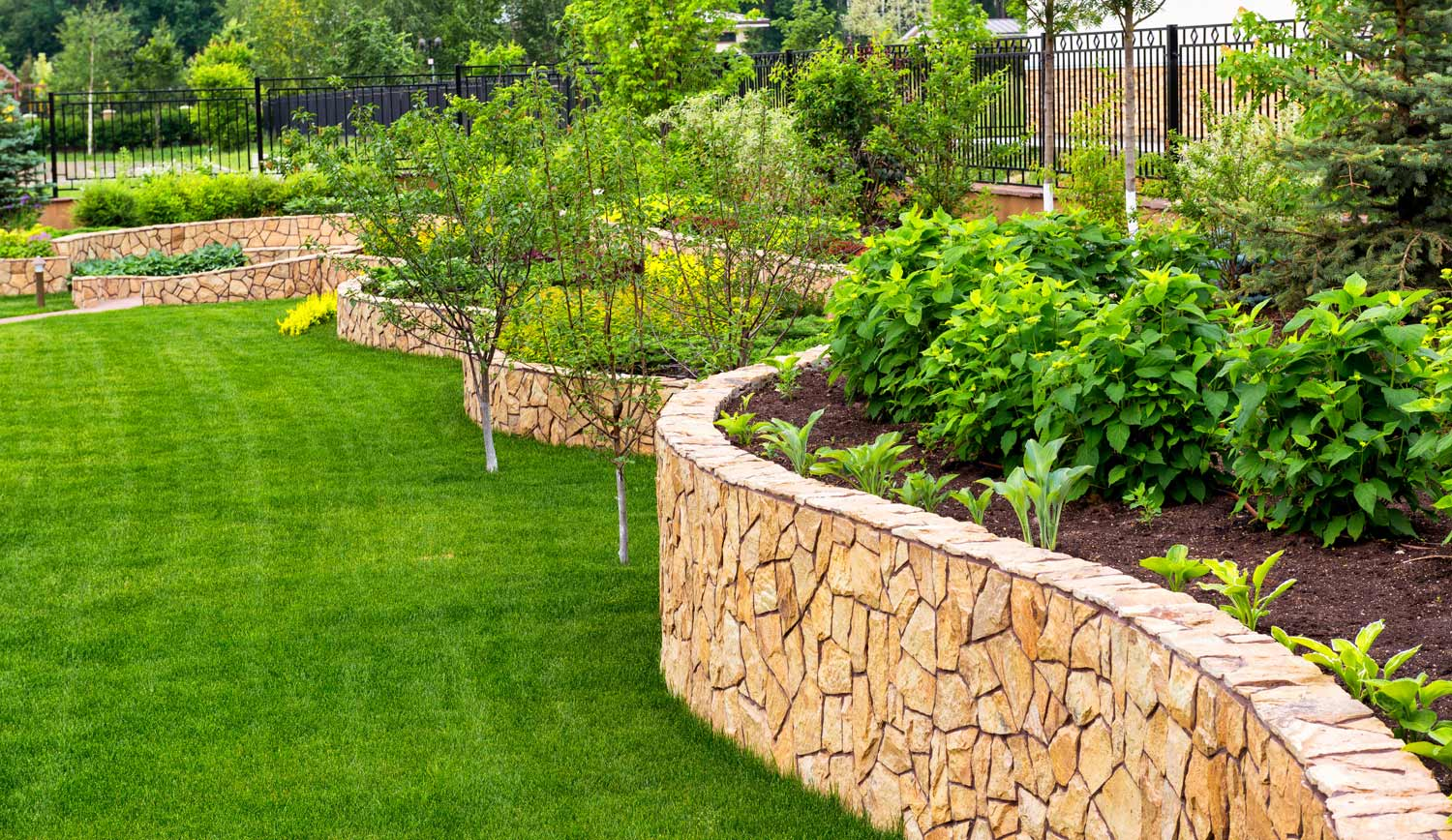Calculate the cubic yard using imperial measurements, metric measurements, or a mix of imperial and metric measurements. Enter inches, feet, yards, centimeters, or meters into the calculator and get the cubic yardage back. This is extremely useful for unit of measurement conversion. One cubic yard is equal to 27 cubic feet (3ft x 3ft x 3ft).

## How to Find Cubic Yardage

Cubic yardage is just a volume, measured in yards. Finding cubic yardage is just like finding a volume, by multiplying the width times the length times the height(W x L x H). For finding the cubic yardage of inches or another unit of measure, first convert the width, length, and height measurements to yards, then multiply.

• Measure the width, length, and height of the volume. If the area is complex, it may be simplest to break the area into manageable parts and calculate the cubic yards of each part separately. For example, if you have a complicated flower bed that you are measuring for mulch, consider breaking it up into geometric areas and then calculate the cubic yardage of each of those areas first, then add them all together.
• Convert these measurements to yards if they aren’t already. Convert inches to yards, convert feet to yards, or convert meters to yards
• Multiply the width times the length times the height (W × L × H) to get the volume in yards.
• If you are calculating yardage for a concrete or landscaping project, understand that suppliers will likely not sell fractions of a yard of material. You may need to round up to the nearest yard and order that amount. It is always worthwhile to ask your supplier if they will sell a partial yard of material, but the majority do not.
• To estimate the cost of your material simply multiply your cubic yardage by the price per yard.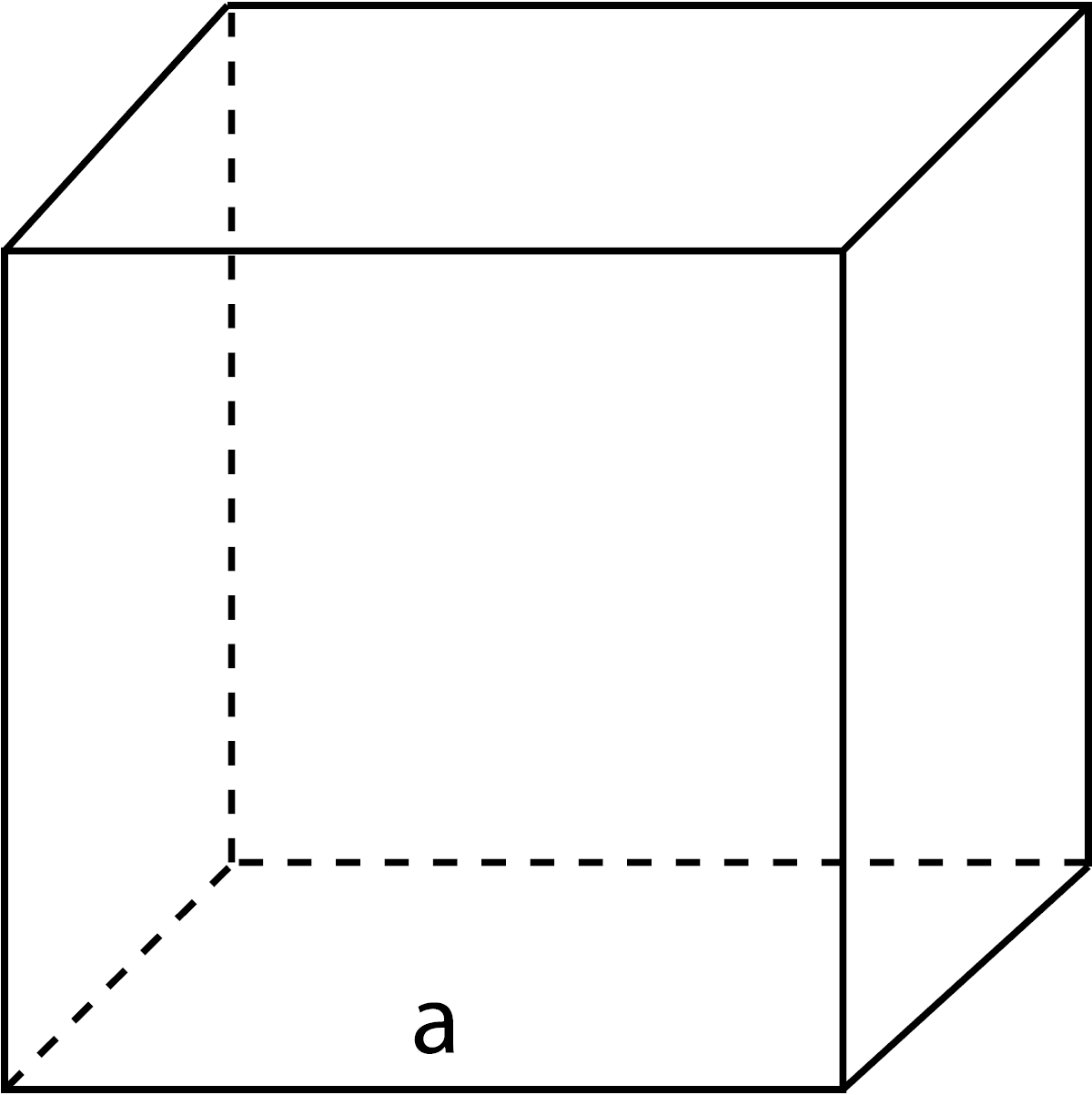### Cube

Calculate the cubic yards of a rectangular cuboid using the formula e3

yds3 = edge x edge x edge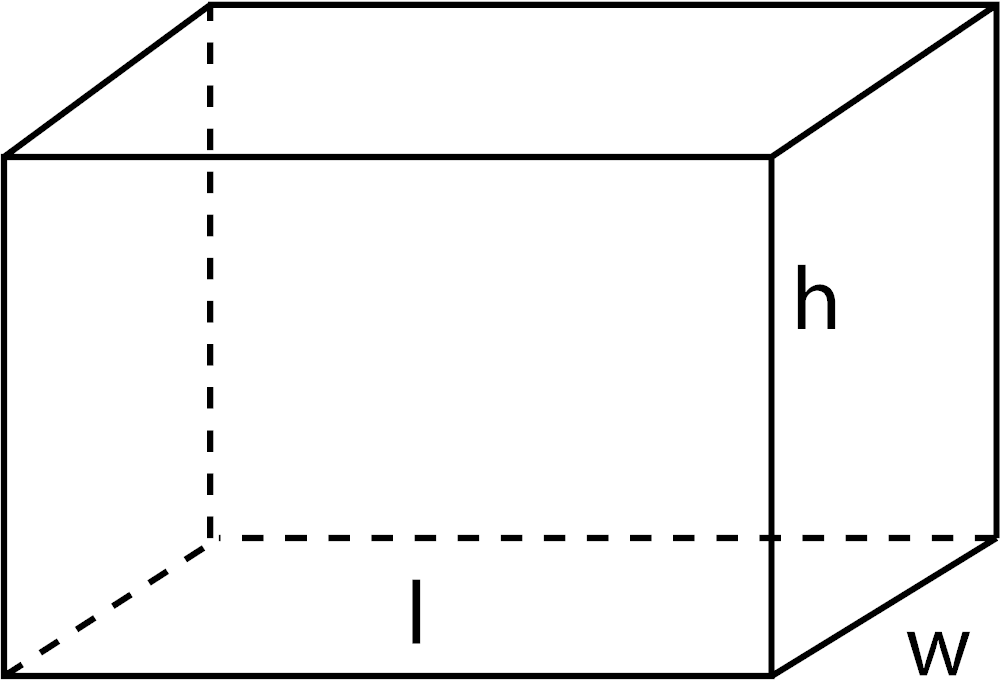### Rectangular Cuboid

Calculate the cubic yards of a rectangular cuboid using the formula w × l × h

yds3 = length × width × height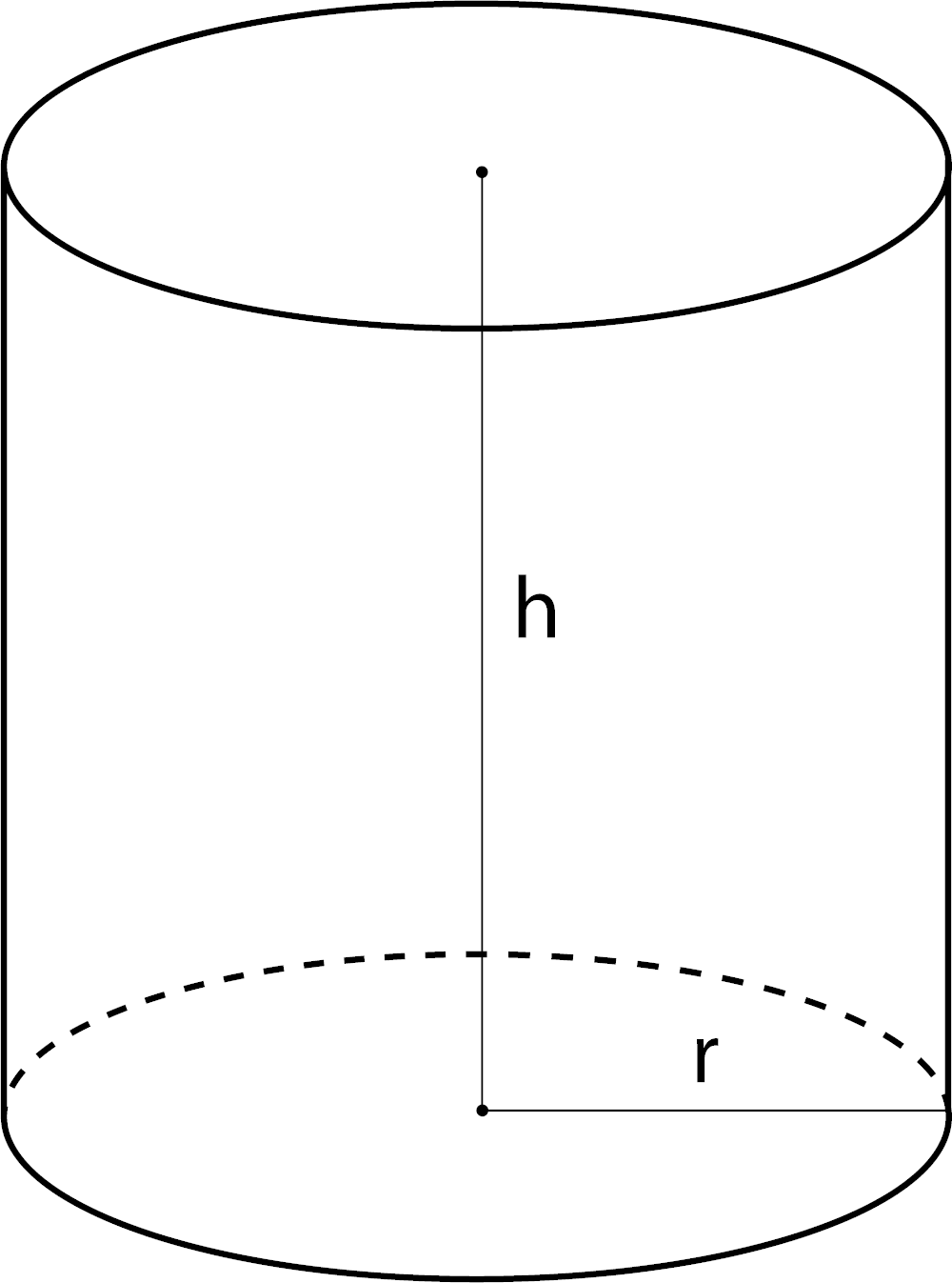### Cylinder Volume

Calculate the cubic yards of a circle or cylinder volume using the formula πr2h

yds3 = ( π × (diameter ÷ 2)2 ) × height
yds3 = π × r2 × h

π = 3.14159265359

Estimate yardage of more shapes using our volume calculator.

## Tips When Calculating Yardage for Landscaping Material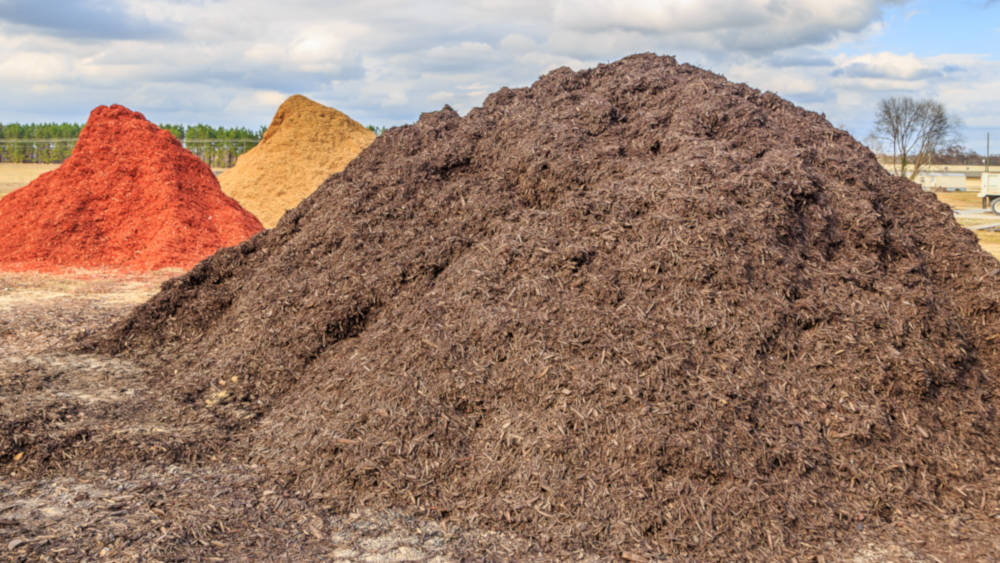Consider buying slightly more material then you estimate you will need if you are calculating yards for landscaping, fill, or concrete. It is always a good idea to have more material than estimated to account for material settling or spilling, and also to compensate for potential mishaps.